# Möbius plane

(diff) ← Older revision | Latest revision (diff) | Newer revision → (diff)
Jump to: navigation, search

circular plane, inversive plane

A plane whose elements form two disjoint sets: the set of points and the set of circles, and which is endowed with a symmetric incidence relation (relating points and circles). The incidence relation satisfies the following axioms:

1) any three distinct points are incident to one and only one circle;

2) given a pointon a circleand a pointnot on, there is a unique circle throughwhose common point withis;

3) there exist at least four distinct points not incident to a circle. Every circle is incident to at least three distinct points.

From a Möbius plane one can obtain an affine plane if one calls one of its points the ideal point, and calls the circles incident to this point straight lines.

In three-dimensional projective spacethe points of an ovoidand the planes that intersect the ovoid at more than one point form, with the incidence relation inherited from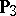, a Möbius plane(see ). A Möbius plane is called egg-like if it is isomorphic to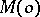for some ovoid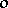. Among the egg-like Möbius planes the best known is the model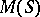, whereis the sphere in three-dimensional Euclidean space, i.e. the plane isomorphic to, whereis a non-ruled quadric in three-dimensional projective space over the field of real numbers.

A Möbius plane is said to be finite if it has a finite number of points and circles. Each circle in a Möbius plane has the same number of points, and through each point of the plane pass the same number of circles. By definition, the order of the plane is the number of points on a circle minus one. A Möbius plane of ordercontains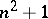points andcircles; through each point of the plane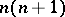circles pass. The following model of the Möbius plane of order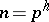is the best known. The points of the plane are the elements of the Galois field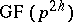and the ideal point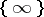; the circles of the plane are the images of the set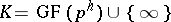under the group of permutations of the form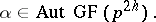A necessary condition for the existence of a Möbius plane of orderis the existence of a finite affine plane of the same order. The uniqueness of the Möbius plane of order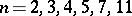has been proven . If a Möbius plane of ordercontains a proper subplane of order, then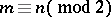and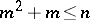(see ).

The classification of Möbius planes has been carried out (see , ). The planes are named after A. Möbius (1855), who laid the foundations of the theory of circles.